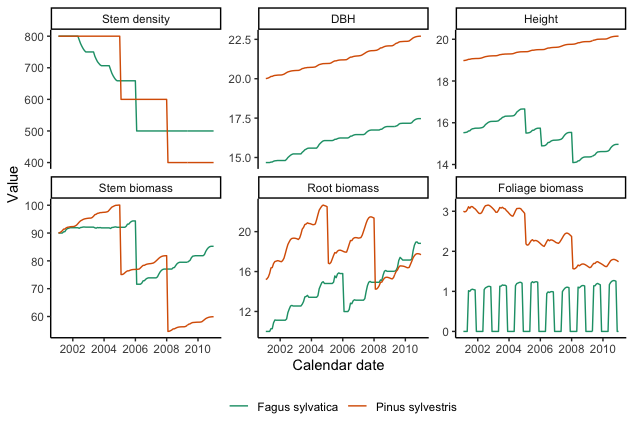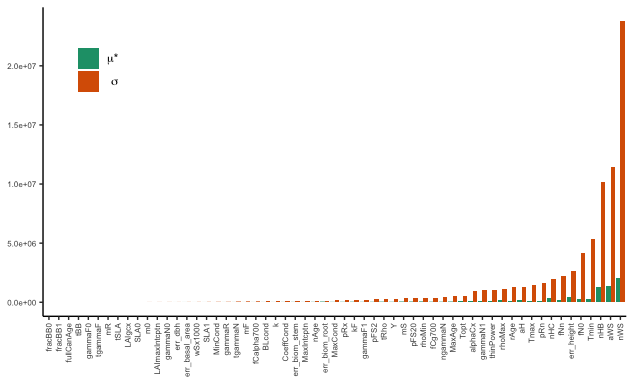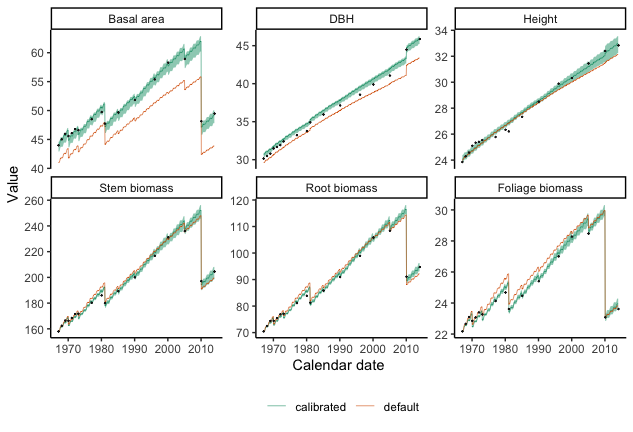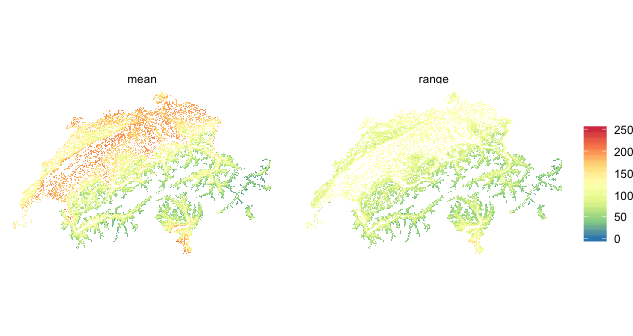# The r3PG R package

#### 2020-06-02

Abstract

This vignette provides an overview of the r3PG R package functions and options. We provide a working examples that (i) demonstrates the basic functionality and use of the package, (ii) performs a sensitivity analysis and a Bayesian calibration of the model, and (iii) uses the calibrated model to simulate spatial patterns of forest growth across Switzerland.

## Purpose

r3PG provides an implementation of the Physiological Processes Predicting Growth (3-PG) model (Landsberg & Waring, 1997). The r3PG serves as a flexible and easy-to-use interface for the 3-PGpjs (Sands, 2010) and the 3-PGmix (Forrester & Tang, 2016) models written in Fortran. The package, allows for fast and easy interaction with the model, and Fortran re-implementation facilitates computationally intensive sensitivity analysis and calibration. The user can flexibly switch between various options and submodules to use the original 3-PGpjs model version for monospecific, even-aged and evergreen forests and the 3-PGmix model, which can also simulate multi-cohort stands (e.g. mixtures, uneven-aged) that contain deciduous species.

## Single model runs

To demonstrate the basic functionality of the r3PG R package, we will perform a simple simulation with the 3-PGmix model. The central function to run 3-PG from R is run_3PG. When called, the function will:

• check the model input for the consistency in structure
• replace the default parameters if new ones are provided
• run and return the simulated output from the model.

Before using run_3PG the user must prepare the input data as described in the help of run_3PG. The inputs include information about site conditions, species initial conditions, climate data, and parameters (if they need to be modified).

In this example, we run a simulation for mixed Fagus sylvatica and Pinus sylvestris stands (Forrester et al., 2017). The input data are provided as internal data in r3PG.

library(r3PG)
library(dplyr)
library(ggplot2)

out_3PG <- run_3PG(
site        = d_site,
species     = d_species,
climate     = d_climate,
thinning    = d_thinning,
parameters  = d_parameters,
size_dist   = d_sizeDist,
settings    = list(light_model = 2, transp_model = 2, phys_model = 2,
height_model = 1, correct_bias = 0, calculate_d13c = 0),
check_input = TRUE, df_out = TRUE)

head( out_3PG )
#>         date         species   group variable      value
#> 1 2001-01-31 Fagus sylvatica climate  tmp_min -1.4669526
#> 2 2001-02-28 Fagus sylvatica climate  tmp_min -0.8616548
#> 3 2001-03-31 Fagus sylvatica climate  tmp_min  3.8046124
#> 4 2001-04-30 Fagus sylvatica climate  tmp_min  2.7947164
#> 5 2001-05-31 Fagus sylvatica climate  tmp_min  9.7551870
#> 6 2001-06-30 Fagus sylvatica climate  tmp_min 10.0666666

The output of the run_3PG function can be either a long format dataframe or a 4-dimentional array where each row corresponds to one month of simulations. The second dimension corresponds to species, where each column represents one species. The third dimension corresponds to variable groups and the variables themselves To get information about output variables and their group, please look at data('i_output').

As an example for how to visualize the output, we select six variables: basal area, dbh, height, stem biomass, foliage biomass, and root biomass. For visualization we are using a ggplot function, which allows us to visualize all the outputs side-by-side.

i_var <- c('stems_n',  'dbh', 'height', 'biom_stem', 'biom_root', 'biom_foliage')
i_lab <- c('Stem density', 'DBH', 'Height', 'Stem biomass', 'Root biomass', 'Foliage biomass')

out_3PG %>%
filter(variable %in% i_var) %>%
mutate(variable = factor(variable, levels = i_var)) %>%
ggplot( aes(date, value))+
geom_line( aes(color = species), size = 0.5)+
facet_wrap( ~ variable, scales = 'free_y', ncol = 3,
labeller = labeller(variable = setNames(i_lab, i_var) )) +
scale_color_brewer('', palette = 'Dark2') +
theme_classic()+
theme(legend.position="bottom")+
xlab("Calendar date") + ylab('Value')## Sensitivity analysis and Bayesian calibration

As a second case study, we want do a slightly more ambitious project, which is a sensitivity analysis and calibration of the model.

For both tasks, we use freely available forest growth data from the PROFOUND database. The data can be extracted from the sql database with the ProfoundData R package, which is available on CRAN. For convenience, however, we have included the extracted and cleaned data in the required format in the r3PG package. All the data used in this vignette can be downloaded from r3PG/vignettes_build

load('vignette_data/solling.rda')

Loading necessary packages

library(tidyr)
library(purrr)
library(BayesianTools)
library(sensitivity)

As a second example, we will first performed a Morris screening and then perform the Bayesian calibration of the 3-PGpjs model. We will use the Solling forest flux site located in Germany at an elevation of 508 m.a.s.l., and dominated by Picea abies. We obtained data for this site via the PROFOUND database that was set up for evaluating vegetation models and simulating climate impacts on forests (Reyer et al., 2019). There are almost 50 years of records for various stand and flux variables for Solling site.

### Likelihood function

To perform a morris sensitivity analysis and Bayesian calibration we construct the log Likelihood function. The log Likelihood function was calculated using normal error assumptions for six variables that describe stand stocks and characteristics: basal area, dbh, height, stem biomass, foliage biomass, and root biomass. To calculate the stand-level stocks, we applied the biomass equations developed for European forests following Forrester et al. (2017) for each measured tree, and summed it up to the stand level in Mg dry matter $$ha^{-1}$$.

r3pg_sim <- function( par_df, ...){
#' @description function to simulate the model for a given parameter
#' @param par_df data frame of the parameters with two columns: parameter, piab

sim.df <- run_3PG(
site = site_solling,
species = species_solling,
climate = climate_solling,
thinning = thinn_solling,
parameters = par_df,
size_dist = NULL,
settings =  list(light_model = 1, transp_model = 1, phys_model = 1),
check_input = TRUE, ...)

return( sim.df )
}

r3pg_ll <- function( par_v ){
#' @param par_v a vector of parameters for the calibration, including errors
#' par_v = par_cal_best

# replace the default values for the selected parameters
err_id = grep('err', par_cal_names)

par_df = data.frame(parameter = par_cal_names[-err_id], piab = par_v[-err_id])
err_v = par_v[err_id]

# simulate the model
sim.df <- r3pg_sim(par_df, df_out = FALSE)
sim.df <- cbind(sim.df[,,2,c(3,5,6)], sim.df[,,4,c(1,2,3)])

# calculate the log likelihood
logpost <- sapply(1:6, function(i) {
likelihoodIidNormal( sim.df[,i], observ_solling_mat[,i], err_def[i] )
}) %>% sum(.)

if(is.nan(logpost) | is.na(logpost) | logpost == 0 ){logpost <- -Inf}

return( logpost )
}

### Priors and default

For Morris sensitivity analysis and Bayesian calibration we define each parameters range, for which we will evaluate the sensitivity and later perform the calibration. In addition, we specified error parameters for the observational data.

# default parameters
par_def <- setNames(param_solling$default, param_solling$param_name)
err_def <- setNames(error_solling$default, error_solling$param_name)

# parameters for calibration and their ranges
param_morris.df <- bind_rows(param_solling, error_solling) %>% filter(!is.na(min))
par_cal_names <- param_morris.df$param_name par_cal_min <- param_morris.df$min
par_cal_max <- param_morris.df$max par_cal_best <- param_morris.df$default

r3pg_ll( par_cal_best)
#>  -191.9541

### Morris sensitivity

Using the settings specified below, we will run a total of 30500 model runs (N (length(factors)+1)) and visualize the results. The morris sensitivity run will take approximately 4 minutes on the working computer.

morris_setup <- createBayesianSetup(
likelihood = r3pg_ll,
prior = createUniformPrior(par_cal_min, par_cal_max, par_cal_best),
names = par_cal_names)

set.seed(432)
morrisOut <- morris(
model = morris_setup$posterior$density,
factors = par_cal_names,
r = 500,
design = list(type = "oat", levels = 20, grid.jump = 3),
binf = par_cal_min,
bsup = par_cal_max,
scale = TRUE)

# summarise the moris output
morrisOut.df <- data.frame(
parameter = par_cal_names,
mu.star = apply(abs(morrisOut$ee), 2, mean, na.rm = T), sigma = apply(morrisOut$ee, 2, sd, na.rm = T)
) %>%
arrange( mu.star )
load('vignette_data/morris.rda')

We visualize the results of the morris analysis by listing all the parameters and error parameters. A high $$\mu^{*}$$ indicates a factor with an important overall influence on model output; a high $$\alpha$$ indicates either a factor interacting with other factors or a factor whose effects are non-linear.

morrisOut.df %>%
gather(variable, value, -parameter) %>%
ggplot(aes(reorder(parameter, value), value, fill = variable), color = NA)+
geom_bar(position = position_dodge(), stat = 'identity') +
scale_fill_brewer("", labels = c('mu.star' = expression(mu * "*"), 'sigma' = expression(sigma)), palette="Dark2") +
theme_classic() +
theme(
axis.text = element_text(size = 6),
axis.text.x = element_text(angle=90, hjust=1, vjust = 0.5),
axis.title = element_blank(),
legend.position = c(0.05 ,0.95),legend.justification = c(0.05,0.95)
)### Bayesian calibration

We will run the Bayesian calibration for the 20 most sensitive parameters defined by the morris sensitivity plus six errors parameters for each of the observational variables. We will run 3 parallel chains, each on a separate computer node for 4e+06 iterations. This can easily be done on a computer server, and takes approximately 21 hours.

# which parameters to calibrate
par_select <- morrisOut.df$parameter %>% .[-grep('err', .)] %>% tail(., 20) %>% as.character() par_id <- which(par_cal_names %in% c(par_select, error_solling$param_name) )

par_cal_names <- par_cal_names[par_id]
par_cal_min <- par_cal_min[par_id]
par_cal_max <- par_cal_max[par_id]
par_cal_best <- par_cal_best[par_id]

mcmc_setup <- createBayesianSetup(
likelihood = r3pg_ll,
prior = createUniformPrior(par_cal_min, par_cal_max, par_cal_best),
names = par_cal_names)
mcmc_out <- runMCMC(
bayesianSetup = mcmc_setup,
sampler = "DEzs",
settings = list(iterations = 4e+03, nrChains = 3))
load('vignette_data/mcmc.rda')

As the first step, we would look at the Gelman-Rubin diagnostic. The convergence can be accepted because the multivariate potential scale reduction factor was $$\le$$ 1.1.

gelmanDiagnostics( mcmc_out )
#> Potential scale reduction factors:
#>
#>                  Point est. Upper C.I.
#> pFS20                  1.01       1.01
#> aWS                    1.01       1.02
#> nWS                    1.01       1.03
#> pRn                    1.02       1.04
#> Tmin                   1.01       1.02
#> Topt                   1.02       1.05
#> Tmax                   1.02       1.03
#> fN0                    1.02       1.03
#> fNn                    1.01       1.02
#> MaxAge                 1.02       1.04
#> rAge                   1.03       1.05
#> gammaN1                1.01       1.01
#> thinPower              1.02       1.05
#> mS                     1.01       1.01
#> alphaCx                1.06       1.12
#> rhoMin                 1.01       1.02
#> rhoMax                 1.01       1.03
#> aH                     1.02       1.04
#> nHB                    1.03       1.06
#> nHC                    1.03       1.06
#> err_basal_area         1.06       1.11
#> err_dbh                1.03       1.05
#> err_height             1.02       1.04
#> err_biom_stem          1.02       1.03
#> err_biom_root          1.02       1.03
#> err_biom_foliage       1.01       1.03
#>
#> Multivariate psrf
#>
#> 1.11

To evaluate the model performance, we draw ~500 samples from the mcmc object and run model simulations for each of the parameter combinations to understand model uncertainties.

par_def.df <- select(param_solling, parameter = param_name, piab = default)

param.draw <- getSample(mcmc_out, numSamples = 500, coda = F, whichParameters = 1:20) %>%
as.data.frame() %>%
mutate(mcmc_id = 1:n()) %>%
nest_legacy(-mcmc_id, .key = 'pars')   %>%
mutate(
pars = map(pars, unlist),
pars = map(pars, ~tibble::enframe(.x, 'parameter', 'piab')),
pars = map(pars, ~bind_rows(.x, filter(par_def.df, !parameter %in% par_cal_names))))

We then simulate forest growth using the defaults and the drawn parameters combinations. Afterwards, we visualize the posteriori prediction range by drawing the $$5^{th}$$ and $$95^{th}$$ range of predictions. The calibrated model simulates the observational data well.

# default run
def_run.df <- r3pg_sim( par_df = par_def.df, df_out = T) %>%
select(date, variable, value) %>%
mutate(run = 'default')

# calibrated run
post_run.df <- param.draw   %>%
mutate( sim = map( pars, ~r3pg_sim(.x, df_out = T)) ) %>%
select(mcmc_id, sim) %>%
unnest_legacy() %>%
group_by(date, variable) %>%
summarise(
q05 = quantile(value, 0.05, na.rm = T),
q95 = quantile(value, 0.95, na.rm = T),
value = quantile(value, 0.5, na.rm = T)) %>%
ungroup() %>%
mutate(run = 'calibrated')

sim.df <- bind_rows( def_run.df, post_run.df)

# Visualize
i_var <- c('basal_area',  'dbh', 'height', 'biom_stem', 'biom_root', 'biom_foliage')
i_lab <- c('Basal area', 'DBH', 'Height', 'Stem biomass', 'Root biomass', 'Foliage biomass')

observ_solling.df <- observ_solling %>%
gather(variable, value, -date) %>%
filter(variable %in% i_var) %>%
filter(!is.na(value)) %>%
mutate(variable = factor(variable, levels = i_var))

sim.df %>%
filter(variable %in% i_var) %>%
mutate(variable = factor(variable, levels = i_var)) %>%
ggplot(aes(date, value))+
geom_ribbon(aes(ymin = q05, ymax = q95, fill = run), alpha = 0.5)+
geom_line( aes(color = run), size = 0.2)+
geom_point( data = observ_solling.df, color = 'grey10', size = 0.1) +
facet_wrap( ~ variable, scales = 'free_y', nrow = 2, labeller = labeller(variable = setNames(i_lab, i_var) )) +
scale_color_manual('', values = c('calibrated' = '#1b9e77', 'default' = '#d95f02')) +
scale_fill_manual('', values = c('calibrated' = '#1b9e77', 'default' = '#d95f02'), guide = F) +
theme_classic() +
theme(legend.position="bottom")+
xlab("Calendar date") + ylab('Value')## Spatial simulations

3-PG is a cohort level model. Thus, to make a simulation across a landscapes, we need to explicitly simulate each individual grid point. For this purpose, we will simulate Picea abies stand biomass across Switzerland on a 1x1 km grid. In total, this sums to ~18’000 grid points over Switzerland.

We have prepared the input data, including climate and soil information for each of the grid points. We used interpolated meteorological data from the Landscape Dynamics group (WSL, Switzerland) based on data from MeteoSwiss stations (Swiss Federal Office of Meteorology and Climatology) by employing the DAYMET method (Thornton, Running, & White, 1997). Grid-specific information on soil type and plant available soil water was retrieved from European Soil Database Derived data (Hiederer 2013). In addition, we use ~500 samples drawn from the previously obtained mcmc object and run model simulations for each of the parameter combinations to understand simulation uncertainties.

load('vignette_data/grid_input.rda')

We construct a function, which requires site and climate information as input, and calculates the $$5^{th}$$, $$50^{th}$$ and $$95^{th}$$ percentile from ~500 simulated runs. To do so, we use the multidplyr package for distributing computing. In total, it takes less than 1 hour on a computer cluster with 50 processes (CPU time ~20 hours).

library(multidplyr)

r3pg_grid <- function(site, forc){
#' @description simulate the n runs for a given site with the drawn parameter combination

r3pg_int <- function( par_df){
#' @description function to run one site and return required output on standing biomass
#' @param par_df a data.frame of parameters

out <- run_3PG(site, species.grid, forc, thinn.grid, par_df, NULL,
list(light_model = 1, transp_model = 1, phys_model = 1), check_input = TRUE,
df_out = F)[,,4,1]

return( last( out ) )
}

site_out <- param.draw %>%
mutate( sim = map( pars, r3pg_int)) %>%
select(mcmc_id, sim) %>%
unnest_legacy() %>%
summarise(
q05 = quantile(sim, 0.05, na.rm = T),
q95 = quantile(sim, 0.95, na.rm = T),
value = quantile(sim, 0.5, na.rm = T))

return( site_out )
}

cl_in <- create_cluster() %>%
cluster_library(c('r3PG', 'purrr', 'dplyr', 'tidyr')) %>%
cluster_copy( r3pg_grid ) %>%
cluster_copy( species.grid ) %>%
cluster_copy( thinn.grid ) %>%
cluster_copy( param.draw )

#' hide the sample_n
sim.grid <- inner_join(site.grid, climate.grid, by = 'grid_id')  %>%
sample_n(10) %>% # remove this for full simulation
partition(grid_id, cluster = cl_in) %>%
mutate( out = map2(site, forc, ~r3pg_grid(.x, .y))) %>%
select( grid_id, out) %>%
collect() %>%
unnest_legacy() %>%
ungroup()
load('vignette_data/grid_sim.rda')

Once the simulations are done, we visualize the results for average standing biomass and associated uncertainties for the whole landscape.

sim.grid %>%
mutate( range = q95 - q05) %>%
select( grid_id, mean = value, range) %>%
gather( variable, value, -grid_id) %>%
inner_join( ., coord.grid, by = 'grid_id') %>%
ggplot( aes(x, y, fill = value) ) +
geom_raster()+
facet_wrap(~variable)+
theme_void()+
coord_equal() +
scale_fill_distiller( '', palette = 'Spectral', limits = c(0, 250))